Next: 4. Numerical Implementation Up: 3. A General TCAD Previous: 3.5 Mechanical Deformation

# 3.6 Model Summary

The developed electromigration model consists of several submodels. The electrical and temperature distribution in the interconnect is determined by the system of equations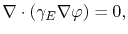(3.71)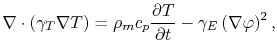(3.72)

with temperature dependent conductivities(3.73)

The vacancy flux is given by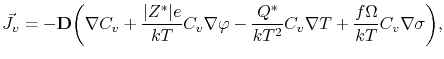(3.74)

which takes into account all driving forces for vacancy transport. The vacancy dynamics is then described by the equations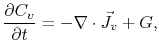(3.75)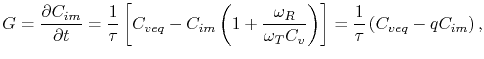(3.76)

where the latter is calculated at grain boundaries and interfaces only.

The trace of the total electromigration strain is calculated by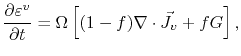(3.77)

which together with (3.75) and (3.76) can be conveniently expressed as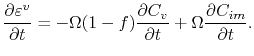(3.78)

The resultant line deformation and mechanical stress is determined by the set of equations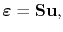(3.79)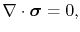(3.80)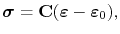(3.81)

where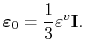(3.82)

The solution of these equations allows a complete cycle of simulation of electromigration in general three-dimensional interconnet structures.Next: 4. Numerical Implementation Up: 3. A General TCAD Previous: 3.5 Mechanical Deformation

R. L. de Orio: Electromigration Modeling and Simulation